Next: The Poisson Equation of Up: Physical Background Previous: Physical Background   Contents

# Basic Equations of Hydrodynamics

The basic equation of hydrodynamics are (1) the continuity equation of the density [equation (A.11)],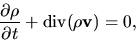(2.1)

(2) the equation of motion [equation (A.7)]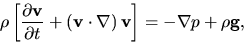(2.2)

and (3) the equation of energy [equation (A.23)]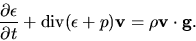(2.3)

Occasionally barotropic relation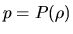substitutes the energy equation (2.3). Especially polytropic relation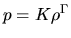is often used on behalf of the energy equation. In the case that the gas is isothermal or isentropic, the polytropic relations of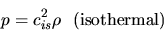(2.4)

and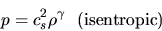(2.5)

are substitution to equation (2.3). [We can replace equation (2.3) with equations (2.4) and (2.5).]Next: The Poisson Equation of Up: Physical Background Previous: Physical Background   Contents
Kohji Tomisaka 2007-07-08